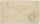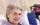How old

The student who asked how many years he answered: "After 10 years I will be twice as old than as I was four years ago. How old is student?

Result

x =  18

Solution:

x+10 = 2* (x-4)

x = 18

x = 18

Calculated by our simple equation calculator.

Leave us a comment of this math problem and its solution (i.e. if it is still somewhat unclear...):

Showing 1 comment:Math student
This may be unclearTo solve this verbal math problem are needed these knowledge from mathematics:

Do you have a linear equation or system of equations and looking for its solution? Or do you have quadratic equation?

Next similar math problems:

1. AuntAnnie aunt is 35 years old, Annie is 14 years. For how many years will aunt have twice as many years as Annie?
2. Mother and daughterMother is 44 years old, her daughter 14. How many years ago was her mother four times older than her daughter?
3. FamilyMom is 39 years old. Karl is eight years and Paul 4 years. For many years mother would old just like two children together?
4. Forest nurseryIn the forest nursery after winter, they found that 1/10 stems died out of them. For them, they land 193 new spruces. How many spruces are in the forest nursery?
5. NormThree workers planted 3555 seedlings of tomatoes in one dey. First worked at the standard norm, the second planted 120 seedlings more and the third 135 seedlings more than the first worker. How many seedlings were standard norm?
6. SuzanSusan's age will be after 12 years four times as much as twelve years ago. How old is Susan now?
7. Bed timeTiffany was 5 years old; her week night bedtime grew by ¼ hour each year. If, at age 18, her curfew time is 11pm, what was her bed time when she was 5 years old?
8. TimeageSeven times of my age is 8 less than the largest two-digit number. How old I am?
9. Unknown number 11That number increased by three equals three times itself?
10. Simple equationSolve the following simple equation: 2. (4x + 3) = 2-5. (1-x)
11. Equation 29Solve next equation: 2 ( 2x + 3 ) = 8 ( 1 - x) -5 ( x -2 )
12. Simple equationSolve for x: 3(x + 2) = x - 18
13. Simple equation 8Solve the following equation: 36=-(1+7x)-6(-7-x)
14. Find xSolve: if 2(x-1)=14, then x= (solve an equation with one unknown)
15. Dropped sheetsThree consecutive sheets dropped from the book. The sum of the numbers on the pages of the dropped sheets is 273. What number has the last page of the dropped sheets?
16. Simple equation 9Solve the following equation: -8y+5=-9y+9
17. Negative in equation2x + 3 + 7x = – 24, what is the value of x?